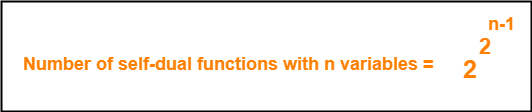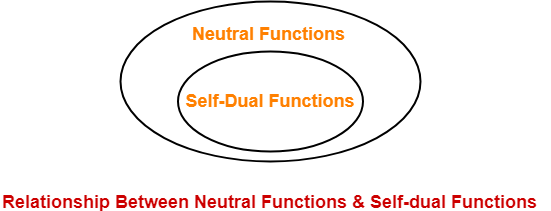## Dual Of Boolean Expression-

To get a dual of any Boolean Expression, replace-

• OR with AND i.e. + with .
• AND with OR i.e. . with +
• 1 with 0
• 0 with 1

### Dual of Boolean Expression Examples-

Following are examples of dual of Boolean Expressions-

#### Example-01:

• Consensus  theorem is xy + x’z + yz = xy + x’z
• Dual of Consensus theorem is (x + y)(x’ + z)(y + z) = (x + y)(x’ + z)

#### Example-02:

• Boolean expression is xyz + x’yz’ + y’z = 1
• Dual of the above Boolean expression is (x + y + z)(x’ + y + z’)(y’ + z) = 0

## Self-Dual Functions-

 When a function is equal to its dual, it is called as a Self dual function.

### Example-

Consider the function : F (A , B , C) = AB + BC + CA

The dual of this function is-

Fd (A , B , C)

= (A + B)(B + C)(C + A)

= AB + BC + CA

Clearly, F (A , B , C) = Fd (A , B , C)

∴ F (A , B , C) is a self-dual function.

## Conditions For Self-Dual Function-

The necessary and sufficient conditions for any function to be a self-dual function are-

• The function must be a Neutral Function.
• The function must not contain any mutually exclusive terms.

### Mutually Exclusive Terms

Consider we have any term X consisting of some variables.

Then, a term obtained by complementing each variable of term X is called as its mutually exclusive term.

### Examples-

• (ABC , A’B’C’) are mutually exclusive terms.
• (AB’C , A’BC’) are mutually exclusive terms.

## Number of Self-Dual Functions-Here n = number of Boolean variables in the function.

### Explanation-

• For a function to be a self-dual function, the function must be a neutral function.
• For a function to be a neutral function, number of minterms must be equal to number of maxterms.
• So, we choose half of the terms i.e. 2n / 2 = 2n-1 terms.
• Now, for each of these terms, we have two choices whether to include it or not in the self-dual function.

So, possible number of self-dual functions

= 2 x 2 x 2 x ……. x 2n-1

= 22^(n-1)

## Relationship Between Neutral Functions & Self-dual Functions-

• Every self-dual function is surely a neutral function.
• But every neutral function need not be a self-dual function.## Important Property of Self-Dual Functions-

 Self-duality is closed under complementation.

### Example-

• If the function F (A , B , C) = ∑ (0 , 1 , 2 , 4) is a self-dual function.
• Then, its complement function F’ (A , B , C) = ∑ (3 , 5 , 6 , 7) will also be a self-dual function.

## Problem-

Consider the following functions-

1. F (A , B , C) = ∑ (0 , 2 , 3)
2. F (A , B , C) = ∑ (0 , 1 , 6 , 7)
3. F (A , B , C) = ∑ (0 , 1 , 2 , 4)
4. F (A , B , C) = ∑ (3 , 5 , 6 , 7)

Which of the above functions are self-dual functions?

1. Only (iii)
2. Only (ii)
3. Only (iii) and (iv)
4. All are self-dual functions

## Solution-

### Condition-01:

According to condition-01, for a function to be a self-dual function, the function must be a neutral function.

• In all the given options, we have functions of 3 variables- A, B and C.
• So, Neutral function must contain exactly 2n-1 = 23-1 = 4 minterms and 4 maxterms.
• But Function-(i) contains only 3 minterms. So, it is not a neutral function.
• Therefore, it can’t be a self-dual function and it gets eliminated.
• We are now left with three other functions which satisfies condition-01 and are all neutral functions.
• We will now use 2nd condition to eliminate the incorrect option(s).

### Condition-02:

According to condition-02, a self-dual function must not contain mutually exclusive terms.

First, let us find which terms are mutually exclusive-

 A B C Minterms 0 0 0 0 A’B’C’ 1 0 0 1 A’B’C 2 0 1 0 A’BC’ 3 0 1 1 A’BC 4 1 0 0 AB’C’ 5 1 0 1 AB’C 6 1 1 0 ABC’ 7 1 1 1 ABC

• From here, pairs of mutually exclusive terms are (0,7) , (1,6) , (2,5) , (3,4).
• Mutually exclusive terms are not allowed in self-dual functions.
• Therefore, terms inside the pairs can not appear together.
• But terms 0 and 7 appear together in the function-(ii).
• So, it can not be a self-dual function.
• But functions (iii) and (iv) do not contain any mutually exclusive terms.
• Therefore, functions (iii) and (iv) are self-dual functions.

Thus, Option (C) is correct.

### NOTE-

• Functions (iii) and (iv) are complementary functions.
• So, if one function is a self-dual function, the other function will also be a self-dual function.
• This is because self-dual functions are closed under complementation.

To gain better understanding about Self-Dual Functions,

Watch this Video Lecture

Get more notes and other study material of Digital Design.

Watch video lectures by visiting our YouTube channel LearnVidFun.